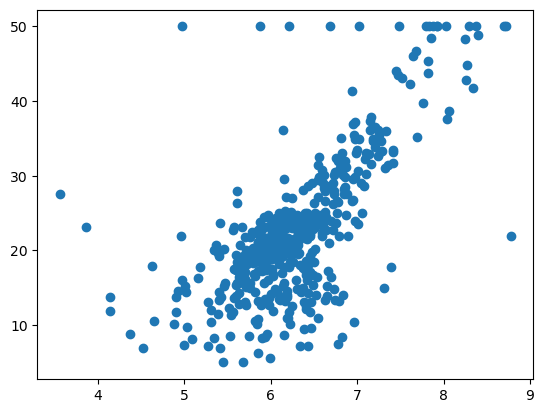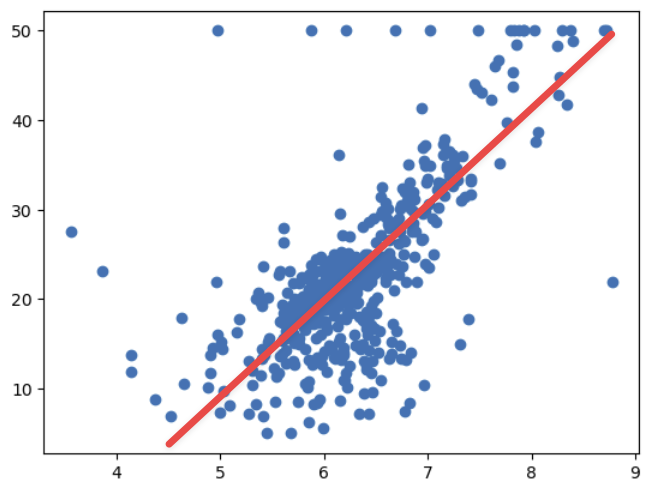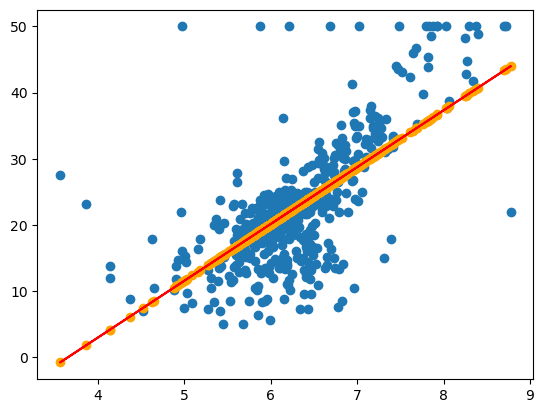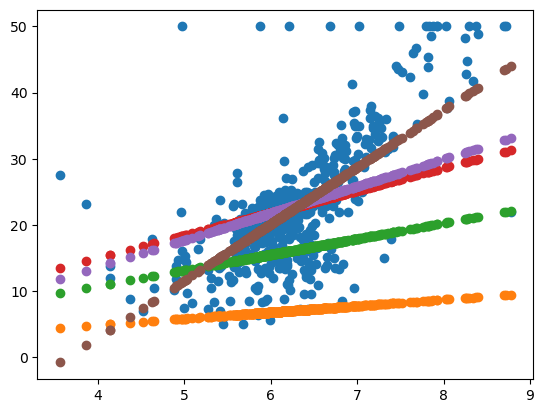# 19. 深度学习 - 用函数解决问题Hi，你好。我是茶桁。$f(x) = k \cdot rm + b$

loss 函数就是在我们进行预测的时候，它的信息损失了多少，所以我们称其为损失函数，loss 函数。 $loss(y, \hat y) = \frac{1}{N}{\sum_{i \in N}}(y_i - \hat {y_i})^2$ y_i - yhat_i 这个值越接近于 0。等于 0 的意思就是每一个预测的 y 都和实际的 y 的值是一样的。那么如果这个值越大指的是预测的 y 和实际的 y 之间差的越大。

$\frac{\partial loss}{\partial k}$

$p' = p + (-1)\frac{\partial loss}{\partial p} * \alpha$

$\alpha$就是一个很小的数，因为我们每次要只能移动很小的一点，不能减小很多。

\begin{align*} k' = k + (-1)\frac{\partial loss}{\partial k} \cdot \alpha \\ b' = b + (-1)\frac{\partial loss}{\partial b} \cdot \alpha \\ \end{align*}

$k_{n+1} = k_n + -1 \cdot \frac{\partial loss(k, b)}{\partial k_n} \\ b_{n+1} = b_n + -1 \cdot \frac{\partial loss(b, b)}{\partial b_n}$19. 深度学习 - 用函数解决问题

https://hivan.me/19. 深度学习 - 用函数解决问题/

Hivan Du

2023-11-10

2023-11-25